# Test: Minors And Cofactors

## 10 Questions MCQ Test Mathematics (Maths) Class 12 | Test: Minors And Cofactors

Description
This mock test of Test: Minors And Cofactors for JEE helps you for every JEE entrance exam. This contains 10 Multiple Choice Questions for JEE Test: Minors And Cofactors (mcq) to study with solutions a complete question bank. The solved questions answers in this Test: Minors And Cofactors quiz give you a good mix of easy questions and tough questions. JEE students definitely take this Test: Minors And Cofactors exercise for a better result in the exam. You can find other Test: Minors And Cofactors extra questions, long questions & short questions for JEE on EduRev as well by searching above.
QUESTION: 1

### Inverse of a matrix A exists, if​

Solution:

Only non-singular matrices have inverses.
Suppose that A is invertible. This means that we have the inverse matrix A−1 of A.
Consider the equation Ax=0. We show that this equation has only zero solution.
Multiplying it by　A−1 on the left, we obtain
A−1 Ax = A-1
⇒ x = 0

QUESTION: 2

Solution:

|kA| = kn|A|
|3A| = (3)n |A|
= 27|A| = k|A|
k = 27

QUESTION: 3

### Find the value of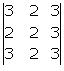Solution:

Explanation:- 3(6-6) -2(6-9) +3(4-6)

= 3(0) + 6 - 6

= 0

QUESTION: 4

If A =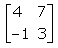, then adj A is:

Solution:
QUESTION: 5

The cofactor of an element 9 of the determinant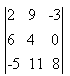is :

Solution:

Co factor of element 9 will be {(6 * 8) -(-5 * 0)}
= 48

QUESTION: 6

The minor Mij of an element aij of a determinant is defined as the value of the determinant obtained after deleting the​

Solution:

A minor, Mij, of the element aij is the determinant of the matrix obtained by deleting the ith row and jth column.

QUESTION: 7

The cofactor of 5 in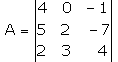Solution:

A21 = {(0, -1) (3,4)}
⇒ (0 - (-3)) = 3
A21 = (-1)(2+1) (3)
= -3

QUESTION: 8

If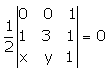, then the relation between x and y is

Solution:

½{(0,0,1) (1,3,1) (x,y,1)} = 0
{(0,0,1) (1,3,1) (x,y,1)} = 0/(½)
{(0,0,1) (1,3,1) (x,y,1)} = 0
0(3-y) -0(1-x) +1(y-3x) = 0
=> y - 3x = 0
=> y = 3x

QUESTION: 9

If matrix A =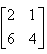and A2 + aA + b = O, then the values of a and b are:

Solution:
QUESTION: 10

If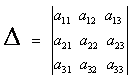and Aij are cofactors of aij, then

Solution:

Δ = Sum of products of element of row(or column)  with their corresponding co-factors.
Δ = a11 A11 + a21 A21 + a31 A31